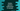# getattr in Python 3 with example## getattr in Python :

getattr is used to get the attribute of an object. We can also pass one default value to print if the attribute is not found.Before going in details, let’s take a look into the following program :

``````class Student():
name = "Albert"
age = "20"
def greet(self,message):
print (message +" "+ self.name)``````

We can create one object of this class and print the values of ‘name’, ‘age’ or call the function ‘greet’. Let’s do that :

``````class Student():
name = "Albert"
age = "20"
def greet(self,message):
print (message +" "+ self.name)

student = Student()

print (student.name)
print (student.age)
student.greet("Hello")``````

Output is :

``````Albert
20
Hello Albert``````

We can also use ‘getattr’ to print these values.

### Syntax of getattr :

Syntax of getattr is :

``getattr(object, name[, default])``

It returns the value of the attribute ‘name’ for ‘object’. ‘default’ is optional. If ‘attribtue’ is not found, it returns the ‘default’ value. One thing we should remember that the ‘attribute’ should be a string value always.

### Using getattr without default value :

We can use ‘getattr’ to print the defined values like below :

``````class Student():
name = "Albert"
age = "20"
def greet(self,message):
print (message +" "+ self.name)

student = Student()

print (getattr(student , "name"))
print (getattr(student , "age"))
getattr(student, "greet")("Hello")``````

Output :

``````Albert
20
Hello Albert``````

### Using getattr with default value :

As stated above, we can also use ‘getattr’ with a default value. If no attribute with the specified ‘key’ is found, it will return the default value. That means, it will not throw any exception . We don’t have any attribute with name as ‘description’ in the above example. Let’s try to request for this attribute with a default value :

``````class Student():
name = "Albert"
age = "20"
def greet(self,message):
print (message +" "+ self.name)

student = Student()

print (getattr(student , "description",'none'))``````

In this example, the output will be ‘none’. We can also use try-except . Let’s take a look :

### Using getattr with try block :

``````class Student():
name = "Albert"
age = "20"
def greet(self,message):
print (message +" "+ self.name)

student = Student()

try:
print (getattr(student , "description"))
except AttributeError:
print ("No attribute found")``````

If you run this code, it will throw an exception ‘AttributeError’ and print the line ‘No Attribute found’.